# AP Physics C: Electricity and Magnetism Practice Test 4

### Test Information10 questions13 minutes

1.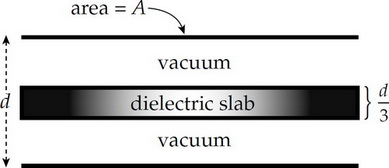A dielectric of thickness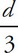is placed between the plates of a parallel-plate capacitor, as shown above. If K is the dielectric constant of the slab, what is the capacitance?

2.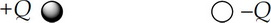Consider the two source charges shown above. At how many points in the plane of the page, in a region around these charges are both the electric field and the electric potential equal to zero?

3.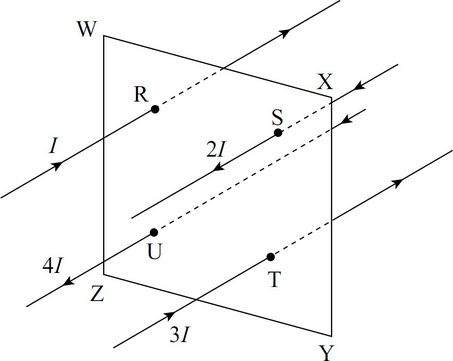The figure above shows four current-carrying wires passing perpendicularly through the interior of a square whose vertices are W, X, Y, and Z. The points where the wires pierce the plane of the square (namely, R, S, T, and U) themselves form the vertices of a square each side of which has half the length of each side of WXYZ. If the currents are as labeled in the figure, what is the absolute value of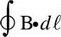where the integral is taken around WXYZ?

4.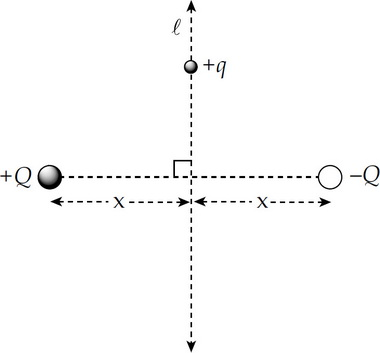The positions of two isolated point charges, +Q and –Q, are fixed as shown in the figure above. Which of the following statements is/are true?

I. A charge +q moving along line 这是啥 would experience no change in electrical potential energy.

II. There is exactly one point on line 这是啥 where the total electrostatic force on +q is equal to zero.

III. At any point P on line 这是啥, the intersection of the equipotential surface containing P with the plane of the figure is an ellipse with the charges +Q and –Q at the foci.

5.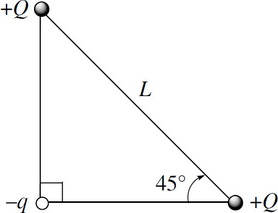Two point charges, each +Q, are fixed a distance L apart. A particle of charge –q and mass m is placed as shown in the figure above. What is this particle's initial acceleration when released from rest?

6.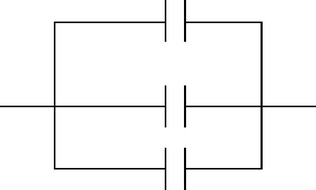Three 3 mF capacitors are connected in parallel as shown above. Determine the equivalent capacitance of the arrangement.

7. A microwave oven is connected to an outlet, 120 V, and draws a current of 2 amps. At what rate is energy being used by the microwave oven?

8.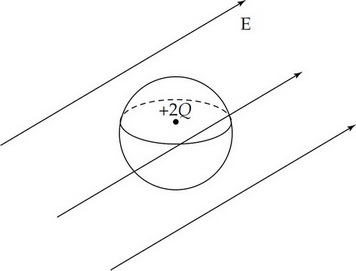A uniform electric field exists in a region, and then a neutral, conducting, spherical shell with a stationary charge +2Q at its center is placed in the region, as shown above. The radius of the sphere is R. The flux through the sphere depends on the value of

9. In a certain region, the electric field varies with the radius away from origin by the equation Er = –6r2 + 4r + 3, where r is given in meters and E in N/C. The potential difference between the origin and the point (3, 4) is

10.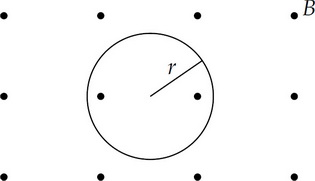A particle of charge –q and mass m moves with speed v perpendicular to a uniform magnetic field B directed out of the page. The path of the particle is a circle of radius r, as shown above.

Which of the following correctly gives the direction of motion and the magnitude of the acceleration of the charge?

Direction &nbps;&nbps;&nbps; Acceleration of Charge